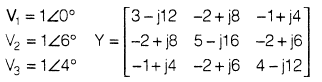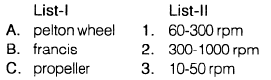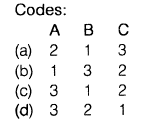# Test: Electrical Machines & Power Systems- 2

## 20 Questions MCQ Test GATE Electrical Engineering (EE) 2023 Mock Test Series | Test: Electrical Machines & Power Systems- 2

Description
Attempt Test: Electrical Machines & Power Systems- 2 | 20 questions in 60 minutes | Mock test for GATE preparation | Free important questions MCQ to study GATE Electrical Engineering (EE) 2023 Mock Test Series for GATE Exam | Download free PDF with solutions
QUESTION: 1

Solution:
QUESTION: 2

Solution:
QUESTION: 3

### Two 50 Hz induction motorshaving 10 & 14 poles are connected in cancated fashion. The possible synchronous speed is ____________

Solution:
QUESTION: 4

A 1φ, 50 Hz induction motor having '6' poles running at 940 rpm. Its slips with respect to backward field and forward fieldrespectively, are_____________

Solution:
QUESTION: 5

A 3φ, 3300 V, Y connected synchronous motor has an effective resistance and synchronous reactance of 2 Ω and 18.0 Ω per phase respectively. IftheO.C. generated emf is 2195 V per phase, the maximumpower developed by motor is______________

Solution:
QUESTION: 6

A line has conductance, inductance, resistance and capacitance as G, L, R & C respectively. Its characteristic impedance is ______________

Solution:
QUESTION: 7

in practice generators are____________

grounded and motors are ______________

grounded

Solution:
QUESTION: 8

A power system consists of 9 buses with 3 machines. Jacobian matrix dimension is

Solution:
QUESTION: 9

For stable operation of power system,synchronous coefficient should be__________

Solution:
QUESTION: 10

A transmission line is connected to a generator, has 0.6 p.u, impedance of 200 MVA & 11 kV base & that of generator is 0.2 p.u. on 100 MVA & 22 kV base. The total p.u. impedance on 500 MVA, 33 kVbase is___________

Solution:
QUESTION: 11

In a 1φ transmission system, the distance between two conductors is 1m and the diameter of each conductor is 4 cm. The net inductance of each conductor is

Solution:
QUESTION: 12

A transmission line has impedance as 200 Ω is terminated with a cable which has impedance as 40 Ω. The coefficient of refraction of current and that of voltagerespectively___________

Solution:
QUESTION: 13

An alternator has positive, negative and zero sequence impedances as j0.25, j0.25 and j0.5 respectively, is grounded with an impedance of j0.2. For a LLG fault on phase ‘b’ and ’c* the positive sequence current ofthe network is____________

Solution:
QUESTION: 14

The Y bus & voltages of a power system given is below. The reactive power of bus 2 is _______________Solution:
QUESTION: 15

On a generator O.C. and S.C tests were conducted and the results are given in below:

O.C. test                         lf =     2.0A

S.C test                           I, =    0.5A

If the rating of generator is 100 MVA, its S.C. MVA is____________

Solution:
QUESTION: 16

A motor receiving 50% of the power that is capable of receiving from an infinite bus. If the load on the motor is increased to 75% of its rating, the maximum value of rotorangle during rotor swinging is_____________

Solution:
QUESTION: 17

An alternator and synchronous motor each rated for 50 MVA, 13.2 kV having subtransient reactance of 20% are connected through a transmission link of 10% on the base of machine ratings. The motor acting as a load of 35 MW at 0.9 p.f. lag and the terminal voltage is 12.5 kV.

Q.

If a 3 phase fault takes place at motor terminals, the subtransient fault current is

Solution:
QUESTION: 18

An alternator and synchronous motor each rated for 50 MVA, 13.2 kV having subtransient reactance of 20% are connected through a transmission link of 10% on the base of machine ratings. The motor acting as a load of 35 MW at 0.9 p.f. lag and the terminal voltage is 12.5 kV.

Q.

In the above problem the subtransientcurrent of motor is____________

Solution:
QUESTION: 19

Match List-I (Hydro turbine) with List-ll (Operating speed) and select the correct answerSolution:
QUESTION: 20

A 3φ transposed transmission line has self impedance of j20 Ω and mutual impedance of j8 Ω. Its zero sequence impedance and positive sequence impedance respectively, are___________

Solution:Use Code STAYHOME200 and get INR 200 additional OFF Use Coupon Code